# Methods and formulas for the shelf life for the mean response for Stability Study for fixed batches

Select the method or formula of your choice.

## Shelf life for the mean response method with only a lower specification limit

To simplify the calculation of the shelf life, consider which model you fit to the data.

### The model with time, batch, and the time*batch interaction

When the batch effect and the batch*time interaction are in the model, the fit for the ith batch at time xij uses the model that follows:

To find the shelf life, set the equation that follows equal to the lower specification limit and solve for time (x).

where

I = the total number of batch levels

n = the total number of response values

X = the design matrix for the model

To calculate a meaningful shelf life, Minitab evaluates two conditions. First, Minitab determines whether the mean response is statistically greater than the lower specification limit at time = 0.

Second, Minitab determines whether the mean response declines at a statistically significant rate over time.

If the initial response is great enough and the response decreases over time, then Minitab calculates the shelf life. To calculate the shelf life, use the quadratic equation as follows:

where

### The model with time and batch

When the batch*time interaction is not in the model, the slopes are the same for every batch. The fit for the ith batch at time xij uses the model that follows:

To find the shelf life, set the equation that follows equal to the lower specification limit and solve for time (x).

where

I = the total number of batch levels

n = the total number of response values

X = the design matrix for the model

To calculate a meaningful shelf life, Minitab evaluates two conditions. First, Minitab determines whether the mean response is statistically greater than the lower specification limit at time = 0.

Second, Minitab determines whether the mean response declines at a statistically significant rate over time.

If the initial response is great enough and the response decreases over time, then Minitab calculates the shelf life. To calculate the shelf life, use the quadratic equation as follows:

where

### The model with time

When only time is in the model, the slopes and intercepts are the same for every batch. The fit at time xij uses the model that follows:

To find the shelf life, set the equation that follows equal to the lower specification limit and solve for time (x).

where

I = the total number of batch levels

n = the total number of response values

X = the design matrix for the model

To calculate a meaningful shelf life, Minitab evaluates two conditions. First, Minitab determines whether the mean response is greater than the lower specification limit at time = 0.

Second, Minitab determines whether the mean response declines over time.

If the initial response is great enough and the response decreases over time, then Minitab calculates the shelf life. To calculate the shelf life, use the quadratic equation as follows: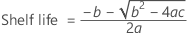where

### Notation

TermDescription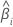the slope for the ith batch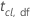the t statistic for the confidence level (cl) and degrees of freedom (df)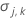the variance of the estimated parameter vector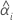the intercept for the ith batch
Lthe lower specification limit
Xthe design matrix
ian index to show the batch that the shelf life estimate is for
lthe number of levels in the batch factor
yijthe response value for the ith batch and the jth time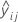the fitted value for the ith batch and the jth time
nthe total number of response values

## Shelf life for the mean response method with only an upper specification limit

To simplify the calculation of the shelf life, consider which model you fit to the data.

### The model with time, batch, and the time*batch interaction

When the batch effect and the batch*time interaction are in the model, the fit for the ith batch at time xij uses the model that follows:

To find the shelf life, set the equation that follows equal to the upper specification limit and solve for time (x).

where

I = the total number of batch levels

n = the total number of response values

X = the design matrix for the model

To calculate a meaningful shelf life, Minitab evaluates two conditions. First, Minitab determines whether the mean response is statistically less than the upper specification limit at time = 0.

Second, Minitab determines whether the mean response increases at a statistically significant rate over time.

If the initial response is low enough and the response increases over time, then Minitab calculates the shelf life. To calculate the shelf life, use the quadratic equation as follows:

where

### The model with time and batch

When the batch*time interaction is not in the model, the slopes are the same for every batch. The fit for the ith batch at time xij uses the model that follows:

To find the shelf life, set the equation that follows equal to the upper specification limit and solve for time (x).

where

I = the total number of batch levels

n = the total number of response values

X = the design matrix for the model

To calculate a meaningful shelf life, Minitab evaluates two conditions. First, Minitab determines whether the mean response is statistically less than the upper specification limit at time = 0.

Second, Minitab determines whether the mean response increases at a statistically significant rate over time.

If the initial response is low enough and the response increases over time, then Minitab calculates the shelf life. To calculate the shelf life, use the quadratic equation as follows:

where

### The model with time

When only time is in the model, the slopes and intercepts are the same for every batch. The fit at time xij uses the model that follows:

To find the shelf life, set the equation that follows equal to the upper specification limit and solve for time (x).

where

I = the total number of batch levels

n = the total number of response values

X = the design matrix for the model

To calculate a meaningful shelf life, Minitab evaluates two conditions. First, Minitab determines whether the mean response is less than the upper specification limit at time = 0.

Second, Minitab determines whether the mean response increases over time.

If the initial response is low enough and the response increases over time, then Minitab calculates the shelf life. To calculate the shelf life, use the quadratic equation as follows:

where

### Notation

TermDescriptionthe slope for the ith batchthe t statistic for the confidence level (cl) and degrees of freedom (df)the variance of the estimated parameter vectorthe intercept for the ith batch
Uthe upper specification limit
Xthe design matrix
ian index to show the batch that the shelf life estimate is for
lthe number of levels in the batch factor
yijthe response value for the ith batch and the jth timethe fitted value for the ith batch and the jth time
nthe total number of response values

## Both limits

To simplify the calculation of the condition for how and when Minitab calculates the shelf life, consider which model you fit to the data.

### The model with time, batch, and the time*batch interaction

Minitab evaluates two conditions to determine whether a meaningful estimate of the shelf life exists. First, Minitab determines whether the mean response is statistically within the specification limits.

where

I = the total number of batch levels

n = the total number of response values

X = the design matrix for the model

Second, Minitab determines whether the mean response changes at a statistically significant rate over time.

If a meaningful estimate exists, then Minitab determines whether the mean response increases or decrease over time. If the second condition is false, then one of the conditions that follows is true.

The response decreases over time.

The response increases over time.

If the mean response decreases over time, then Minitab calculates the shelf life relative to the lower specification limit. Otherwise, Minitab calculates the shelf life relative to the upper specification limit.

### The model with time and batch

Minitab evaluates two conditions to determine whether a meaningful estimate of the shelf life exists. First, Minitab determines whether the mean response is statistically within the specification limits.

where

I = the total number of batch levels

n = the total number of response values

X = the design matrix for the model

Second, Minitab determines whether the mean response changes at a statistically significant rate over time.

If a meaningful estimate exists, then Minitab determines whether the mean response increases or decrease over time. If the second condition is false, then one of the conditions that follows is true:

The response decreases over time.

The response increase over time.

If the mean response decreases over time, then Minitab calculates the shelf life relative to the lower specification limit. Otherwise, Minitab calculates the shelf life relative to the upper specification limit.

### The model with time

Minitab evaluates two conditions to determine whether a meaningful estimate of the shelf life exists. First, Minitab determines whether the mean response is statistically within the specification limits.

where

I = the total number of batch levels

n = the total number of response values

X = the design matrix for the model

Second, Minitab determines whether the mean response changes at a statistically significant rate over time.

If a meaningful estimate exists, then Minitab determines whether the mean response increases or decrease over time. If the second condition is false, then one of the conditions that follows is true:

The response decreases over time.

The response increases over time.

If the mean response decreases over time, then Minitab calculates the shelf life relative to the lower specification limit. Otherwise, Minitab calculates the shelf life relative to the upper specification limit.

### Notation

TermDescriptionthe slope for the ith batch
lthe number of levels in the batch factor
nthe number of rows in the datathe value of the inverse cumulative distribution at cl from the t distribution with df degrees of freedom
By using this site you agree to the use of cookies for analytics and personalized content.  Read our policy